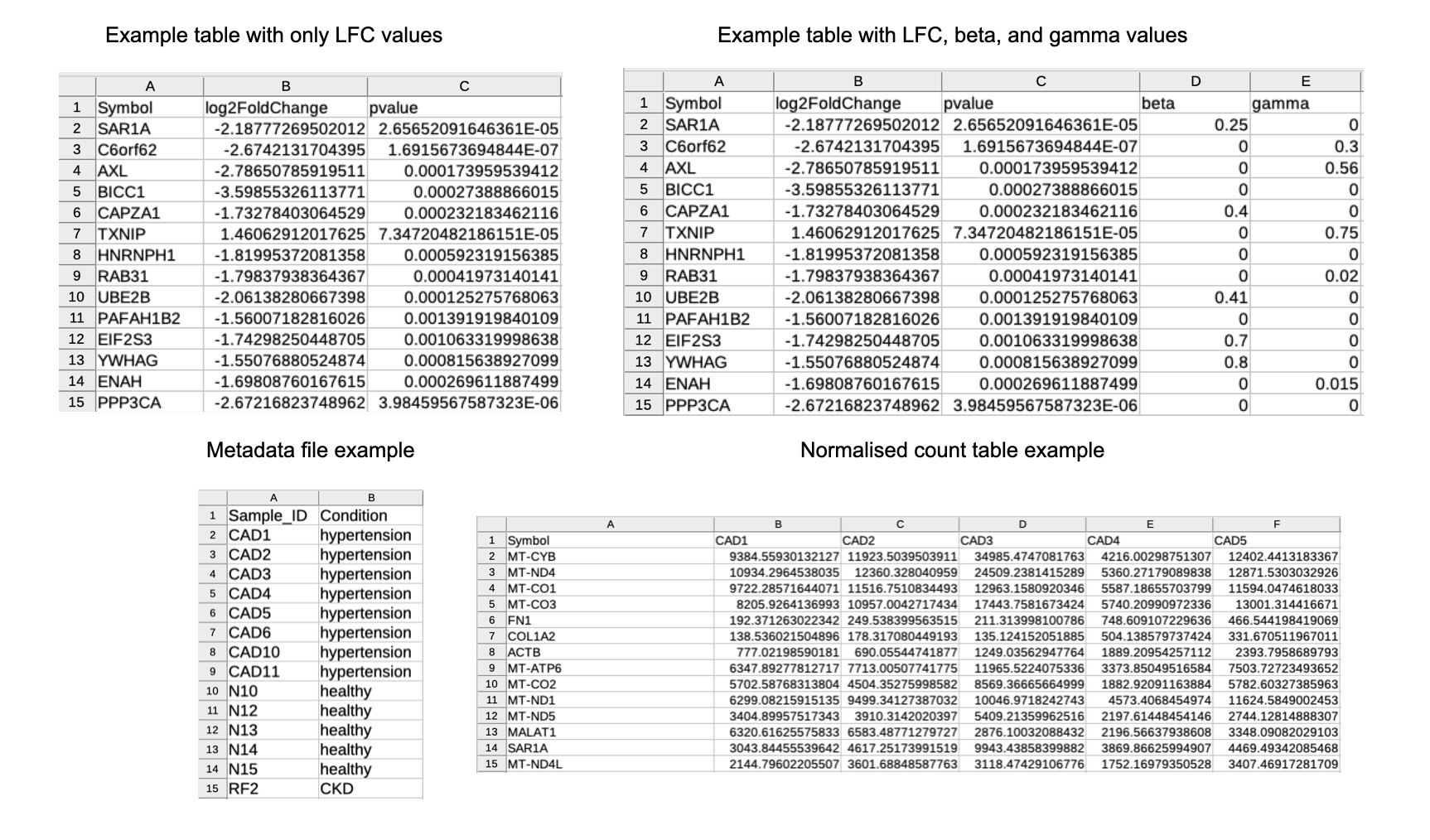OmicInt Package: exploring omics data and regulatory networks using integrative analyses and machine learning

library(OmicInt)

1. Package Overview

OmicInt is an R package developed for an in-depth exploration of significantly changed genes, gene expression patterns, and associated epigenetic features as well as miRNA environment. The package helps to assess gene clusters based on their known interactors (proteome level) using several different resources, e.g. UniProt  and STRINGdb . Moreover, OmicInt provides an easy Gaussian mixture modelling (GMM) [3,4] pipeline for an integrative analysis that can be used by a non-expert to explore gene expression data. Specifically, the package builds on a previously developed method to explore gene networks using significantly changed genes, their log-fold-change values (LFC), and the predicted interactome complexity . This approach can aid in studying specific gene networks, understanding cellular perturbation events, and exploring interactions that might not be easily detectable otherwise . To this end, the package offers many different utilities to help researchers quickly explore their data in a user-friendly way where machine learning is made easily accessible to non-experts (Fig.1&2).

Before starting the analysis the user must ensure that the supplied data is in the right format. There are several different options to prepare a data frame (CSV format) that contains all relevant experimental information (Fig.1; Eq.1-4). Depending on the selection, the downstream analyses will provide interactive graphs and maps (Fig.2).

The key analytical parameter in the machine learning pipeline and exploratory analyses is a specific score, namely $$LFC_{score}$$, which can have a different derivation depending on the selected parameters (Eq.1-3). The user has several options to select from since the equations were expanded with additional data based on the earlier multi-omics equation . The score $$\alpha$$ values are downloaded automatically from curated database images via text mining to retrieve, update, and integrate data in an easier format (i.e., database image) for the analyses. Databases used include Disgenet , Uniprot , and STRINGDB . For example, $$\alpha_{asoc}$$ score describes how a gene associates with a specific pathology based on different curated resources as described earlier . This value allows to infer how strongly a gene is linked to a disease or pathological phenotype ranging from 0 (no link) to 1 (the strongest association) (Eq.1). Similarly, $$\alpha_{spec}$$ captures how specific a gene is when describing the pathology (Eq.2). This is defined by the user as either “association_score” or “specificity_score” when selecting the type of the equation for $$LFC_{score}$$. Scores $$\beta_{cell}$$ and $$\gamma_{prot}$$ are scaled values for single cell and proteome data, respectively. That is, $$\beta_{cell}$$ has to be provided by the user if they have such experimental information integrated where a gene value from a single cell data cluster is extracted using a pseudo-bulk differential gene expression approach. The LFC scores from pseudo-bulk data need to be scaled according to the equation 4. The same approach should be applied when calculating $$\gamma_{prot}$$ for protein (corresponding gene) values.

$$LFC_{score}=LFC(1+\alpha_{asoc}+\beta_{cell}+\gamma_{prot})$$

Equation 1. $$LFC_{score}$$ equation where LFC - Log Fold Change, base 2; $$\alpha_{asoc}$$ - disease association score; $$\beta_{cell}$$ - scaled single cell LFC; $$\gamma_{prot}$$ - scaled proteome LFC.

$$LFC_{score}=LFC(1+\alpha_{spec}+\beta_{cell}+\gamma_{prot})$$

Equation 3. $$LFC_{score}$$ equation where LFC - Log Fold Change, base 2; $$\alpha_{spec}$$ - disease specificity score; $$\beta_{cell}$$ - scaled single cell LFC; $$\gamma_{prot}$$ - scaled proteome LFC.

$$LFC_{score}=LFC(1+\sqrt{\alpha_{asoc} \cdot \alpha_{spec}}+\beta_{cell}+\gamma_{prot})$$

Equation 3. $$LFC_{score}$$ equation where LFC - Log Fold Change, base 2; $$\alpha_{asoc}$$ and $$\alpha_{spec}$$ are integrated using geometric average score; $$\beta_{cell}$$ - scaled single cell LFC; $$\gamma_{prot}$$ - scaled proteome LFC.

$$LFC_{scaled}=\frac{LFC_{gene}}{LFC_{median}}$$

Equation 4. $$\beta_{cell}$$ or $$\gamma_{prot}$$ scaling example where $$LFC_{gene}$$ - gene specific value and $$LFC_{median}$$ - median value for all available LFC per specific condition and gene set.

OmicInt provides many other valuable tools to map interactome using information on the target cellular location or protein class/functional type. In addition, density functions allow for an in-depth assessment of gene distributions which may hint at potential functions or dominating processes within a specific condition. Epigenetic feature (CpG islands, GC%) and miRNA exploration tools also provide additional information on the epigenome and non-coding regulome which might be relevant for some genes and conditions, especially if a higher enrichment of these patterns can be found.
Currently, the analyses are only available for Human data sets.

2.1. Preprocessing

Data pre-processing relies on the score_genes function that collects data from STRINGDB  and disease association databases to scale and prepare additional score integration. Several key parameters should be provided; data parameter requires a data frame containing gene names as row names and a column with LFC values. The example is provided in Figure 1; parameter alpha has a default value set as “association” which gives a score from 0 to 1 based on how strongly a gene is associated with a pathological phenotype; other options are “specificity” - to give values based on how specific a gene is when describing a disease and “geometric” - to give a geometric mean score of both association and specificity. In addition, it is possible to add weighed single cell and proteomics data by selecting additional parameters. Parameter beta is set to have a default value as FALSE; if TRUE, please supply data with a column beta that contains information on gene associations from single cell studies. Similarly, parameter gamma has a default value FALSE; if TRUE, the user needs to supply data with a column gamma that contains information on gene associations from proteome studies.

The function returns a data frame for the downstream analyses.